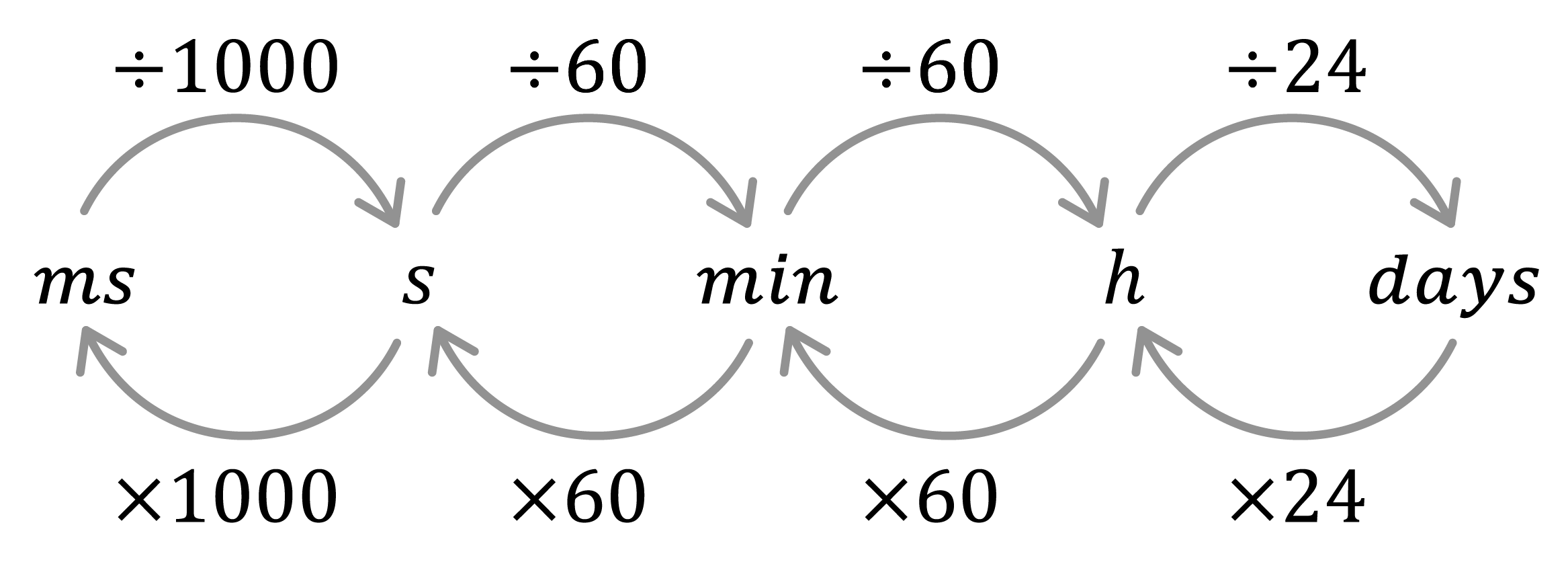Chapter overviewMaths

Exam board

AQA

Number

Algebra

Graphs

Ratio proportion and rates of change

Shapes and area

Angles and geometry

Trigonometry

Probability

Statistics

Maths

# Time intervals: converting units of time0%

Summary

# Time intervals: converting units of time

## ​​​​In a nutshell

Just like with converting between units of mass, length and volume, units of time often need to be converted between; especially when working with time intervals.

## Converting time units

The following conversion diagram can be used to convert between units of time. It's important that you learn these off by heart!​​​$ms$​ - milliseconds$s$​ - seconds $min$ - minutes$h$​ - hoursdays

##### Example 1

How many minutes are there in $36$ hours?​

Work out the conversion factor.

$60 \text{ minutes} = 1 \text{ hour}$

The conversion factor is $60$.

To go from hours to minutes, multiply.

$36\times60=\underline{2160}$ minutes

### Converting time units in steps

Sometimes a conversion requires more than one step.

##### Example 2

How many seconds are there in 6 days?

First convert six days into hours.

$6\times24=144$ hours

Then convert into minutes.

$144\times60=8640$ minutes

Then finally convert into seconds.

$8640 \times60=\underline{518400}$ seconds​

### Conversions involving decimals

When decimals are involved in time conversions, care must be taken. When working with two time units, always make sure to separate the integers (whole numbers) and the decimals, converting the decimals into the smaller unit.

#### PROCEDURE

 1 Divide by the conversion factor to get a decimal. 2 Multiply the whole number by the conversion factor and take this away from the original time. 3 Use what's left as the smaller units and write the answer using both units.

##### Example 3

What is $728$ hours in days and hours?

Divide by the conversion factor: $24$.

$728\div24=30.3333$

Multiply the whole number (thirty) by the conversion factor and take away from $728$.

$30\times24=720\\728-720=8$

Write the answer using what's left as the smaller units.

$\underline{30}$ days and $\underline8$ hours

Conversion of time units are often used when answering questions about timetables. Timetables are written in chronological order, meaning as you read across, the times increase.

##### Example 4

The timetable is part of a bus timetable showing departures from three different stops.

 Stop 1 ​$15:21$​​ ​$15:53$​​ ​$16:25$​​ $16:57$​​ Stop 2 ​$15:26$ ​​$16:00$​ ​$16:32$​​​ ​​$17:04$​ Stop 3 ​​$15:30$​ ​​$16:04$​ ​​$16:36$​ ​​$17:08$​

How long is there between the first and last bus from any stop? Give your answer in minutes.

First notice that at each stop, the bus comes equally as often. Therefore, only one row needs to be focused on - let's use 'Stop 1'.

Read along from 'Stop 1' to the first and last column to identify the first and last bus times.

First: $15:21$​​

Last: $16:57$​​

Work out the difference between the two times in steps.

$15:21 \rightarrow 16:21=1$ hour

$16:21 \rightarrow 16:57 = 36$ minutes

$1$​ hour $36$​ minutes
$1$ hour $= 60$ minutes
$1$ hour $36$ minutes $= 60 +36 = \underline{96}$ minutes